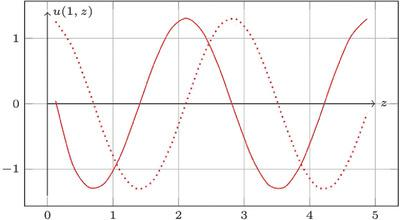ZAMM - Journal of Applied Mathematics and Mechanics ( IF 1.103 ) Pub Date : 2020-01-20 , DOI: 10.1002/zamm.201900170
Alexander O. Vatulyan; Pavel S. Uglich; Oksana V. YavruyanAn effective technique for inverse coefficient problem solving of the shear modulus reconstruction for both inhomogeneous elastic and viscoelastic cylindrical waveguide is proposed. The identification is carried out using the displacement field data measured on the finite part of the outer boundary of the cylindrical waveguide in the torsional vibrations mode. An iterative scheme for solving the inverse problem is developed. At each iteration step, the correction to the shear modulus distribution function is calculated using the Fredholm integral equation of the first kind with a smooth kernel. Numerical results are obtained for both direct and inverse problems for different laws of the shear modulus variation.

down
wechat
bug